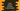# Java program to check if a number is a duck number or not## Java program to check if a number is a duck number or not:

In this post, we will learn how to check if a number is a duck number or not in Java. We will write one program that will take a number as input from the user and print if it is a duck number or not.

### What is a duck number:

A number is called a duck number if it contains at least one zero and if it is a positive number and it is not started with 0.

For example,

• 1234 is not a duck number because there is no zero in it.
• 12304 is a duck number because there is one zero.
• 01204 is not a duck number because it starts with a zero.
• 120034 is a duck number because it has two 0

### Algorithm to check if a number is duck number or not:

We will use the below algorithm to check if a number is duck number or not:

• Read the number as string and store it in a string variable
• Check if the first character is zero or not. If yes, it is not a duck number.
• If the first character is not zero, check all other characters one by one.

• If any of the characters is 0, it is a duck number.
• Else, it is not a duck number.

### Method 1: Java program to check if a number is duck number or not:

Let’s write a Java program to check if a number is a duck number or not:

``````import java.util.Scanner;

public class Main {

public static void main(String[] args) {
String num;
boolean isDuck;
Scanner sc = new Scanner(System.in);

System.out.println("Enter a number: ");
num = sc.nextLine();

if (num.charAt(0) == '0') {
isDuck = false;
} else {
isDuck = false;
for (int i = 1; i < num.length(); i++) {
if (num.charAt(i) == '0') {
isDuck = true;
break;
}
}
}

if (isDuck) {
System.out.println("It is a duck number");
} else {
System.out.println("It is not a duck number");
}

}
}``````

Here,

• num is a string variable to hold the number. isDuck is a boolean value to define if the current number is duck or not.
• It asks the user to enter a number, read it and store it in num.
• If the first character is 0, it assigns false to isDuck.
• If the first character is not 0,

• It assigns isDuck to false first and,
• By using a for loop, it iterates through other characters from index 1 to end.
• If it finds any character equal to 0, it marks isDuck to true and breaks from the loop.
• At the end of the program, it checks the value of isDuck and prints one message based on it.

If you run this program, it will print output as like below:

``````Enter a number:
01234
It is not a duck number

Enter a number:
1234009890
It is a duck number

Enter a number:
1234
It is not a duck number

Enter a number:
012309
It is not a duck number``````

### Method 2: Java program to check if a number is duck number or not by using a different method:

We can also use a different method to check if a number is a duck number or not. This method will take the number as the parameter and return one boolean value, true if it is a duck number and false if it is not.

Let me show you the full program:

``````import java.util.Scanner;

public class Main {

public static boolean isDuck(String no) {
if (no.charAt(0) == '0') {
return false;
}

for (int i = 1; i < no.length(); i++) {
if (no.charAt(i) == '0') {
return true;
}
}

return false;
}

public static void main(String[] args) {
String num;
Scanner sc = new Scanner(System.in);

System.out.println("Enter a number: ");
num = sc.nextLine();

if (isDuck(num)) {
System.out.println("It is a duck number");
} else {
System.out.println("It is not a duck number");
}

}
}``````

In this program,

• isDuck is the method used to check if a number is a duck number or not.

• It takes one String as the input and returns one boolean value indicating the number is a duck or not.
• The advantage of using a separate method is that we can call this method from any other file as well.
• Inside this method,

• It first checks if the first character is 0 or not. If yes, it returns false, i.e. it is not a duck number.
• It iterates through the characters of the string starting from the second character. If it finds any character which is equal to 0, it returns true, i.e. it is a duck number.
• If the loop ends, i.e. if no character is 0 in that string, it returns false.

If you run this program, it will give similar output.